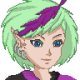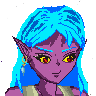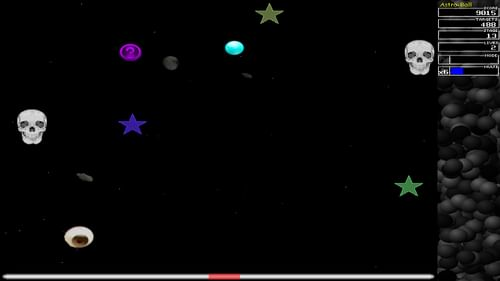-=+=- -=+=- -=+=- -=+=- -=+=- -=+=- -=+=- -=+=- -=+=- -=+=- -=+=- -=+=- -=+=- -=+=- -=+=- -=+=- -=+=- -=+=- -=+=- -=+=- -=+=- -=+=- -=+=- -=+=- -=+=- -=+=- -=+=- -=+=- -=+=- -=+=- (c) WidthPadding Industries 1987 0|420|0 -=+=- -=+=- -=+=- -=+=- -=+=- -=+=- -=+=- -=+=- -=+=- -=+=- -=+=- -=+=- -=+=- -=+=- -=+=- -=+=- -=+=- -=+=- -=+=- -=+=- -=+=- -=+=- -=+=- -=+=- -=+=- -=+=- -=+=- -=+=- -=+=- -=+=-### Tricky

View Profile, Send Private Message
Recent Posts, Showcases, Articles, Code Snippets, Links, Wed.Workshop, Blog

### Latest Showcases

Tricky# Kthura - Map Editor

24th October 2019Let's show you my Kthura Map Editor

[img]https://user-images.githubusercontent.com/11202073/67527190-b6087880-f6b6-11e9-8fdd-c2a2b47011b3.png[/img]

Kthura is a map system for 2D Map development, but here's the rub... It's not a tile s*snip*

Tricky# Astrobounce

18th October 2019Astrobounce